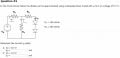# Solving Simple Circuit Involving Diodes (part 1)

Thread Starter

#### Student01

Joined Apr 15, 2009
35
Hey all,

I'm stuck on the following question. Can I combine the two diodes to make a single one, with (0.7 + 0.7=)1.4 V? And how do I go about solving once/if I've done that?#### kiranag

Joined Apr 14, 2009
12
Yes. You can certainly combine two diodes and use 1.4v for all your calculation purpose. Let the voltage at the node be V1 and current flowing through the resistor R1 be I1. V1=I1*R1. Let the current flowing through the main circuit be I. By applying two KVL equations to the two meshes which include the source and putting I as I1+Id, we obtain the relation between Vs and Id as Id=(Vs+5.6)/300. Got it?Thread Starter

#### Student01

Joined Apr 15, 2009
35
Thanks, kiranag, this helps a lot.

Could you possibly tell me if you could also solve this circuit by finding the Thevenin Equivalent of the linear half of the circuit, and then use KVL to find Id? This is how I was attempting to solve it, and how I think I've seen similar problems solved. But I get a different answer. I want to know if this method is not applicable, or if I'm making an error along the way some where.

#### hgmjr

Joined Jan 28, 2005
9,029
Could you possibly tell me if you could also solve this circuit by finding the Thevenin Equivalent of the linear half of the circuit, and then use KVL to find Id? This is how I was attempting to solve it, and how I think I've seen similar problems solved. But I get a different answer. I want to know if this method is not applicable, or if I'm making an error along the way some where.
Thevenin's Method can be applied to the resistive divider R2(leftmost) and R1 to get the Vth and Rth.

If you have tried this approach and encountered a problem then I suggest you post your work and let us see where you got off the track.

hgmjr

#### PRS

Joined Aug 24, 2008
989
The simplest way to solve it is to write a KCL equation at the central node.

(V-Vs)/R2 + (V/R1) + (V-1.4)/R2 = 0

Knowing V you can now find Id = (V-1.4)/R2

Thread Starter

#### Student01

Joined Apr 15, 2009
35
Thanks, PRS, I remember trying this approach before and running into a snag somewhere along the way. I can't remember what went wrong, but this seems self explanatory.

Ok, for the Thevenin Equivalent cirtcuit I get:
R = (300x100)/(300 + 100) + 300 = 375Ω
V = 8.7/300 x 375 = 10.15 V

I = (10.15 - 1.4) / 375 = 0.0233 A

Yet, when I use the method PRS has detailed, I get:

(V1-8.7)/300 + V1/100 + (V1-1.4)/300 = 0
V1 -8.7 + 3V1 + V1 - 1.4 = 0
5V1 = 10.1
V1 = 2.02 V
Id = (2.02 -1.4)/300 = 0.002067 A

Where am I going wrong?

#### t_n_k

Joined Mar 6, 2009
5,455
Your assumptions for the Thevenin equivalent voltage are incorrect. It should "trouble" you that (in the absence of any controlled sources) you get a Thevenin voltage greater than the original source voltage. Work out the Thevenin equivalent for just those elements comprising the source and the two left hand R1 & R2 values. Then use that Thevenin eqivalent to feed the right hand R2 and the two diodes.

Id = 0.002067A is the correct value.

Thread Starter

#### Student01

Joined Apr 15, 2009
35
It should "trouble" you that (in the absence of any controlled sources) you get a Thevenin voltage greater than the original source voltage.
I did notice and wonder about that. :/

I see where I was going wrong now. It should have been:

R = (300x100)/(300 + 100) + 300 = 375Ω
V = 8.7/300 x 75 = 2.175 V

I = (2.175 - 1.4) / 375 = 0.00206 A
Thanks so much for your help, t_n_k, I think I'm finally beginning to get this stuff.Similar threads